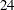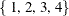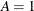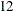### Example 3.2 Alphabet Blocks Problem

This example illustrates usage of the global cardinality constraint (GCC). The alphabet blocks problem consists of finding an arrangement of letters on four alphabet blocks. Each alphabet block has a single letter on each of its six sides. Collectively, the four blocks contain every letter of the alphabet except Q and Z. By arranging the blocks in various ways, the following words should be spelled out: BAKE, ONYX, ECHO, OVAL, GIRD, SMUG, JUMP, TORN, LUCK, VINY, LUSH, and WRAP.

You can formulate this problem as a CSP by representing each of theletters with an integer variable. The domain of each variable is the setthat represents block1 through block4. The assignment '' indicates that the letter 'A' is on a side of block1. Each block has six sides; hence each valueinhas to be assigned to exactly six variables so that each side of a block has a letter on it. This restriction can be formulated as a global cardinality constraint over allvariables with common lower and upper bounds set equal to six.

Moreover, in order to spell all of the words listed previously, the four letters in each of thewords have to be on different blocks. Another GCC statement that specifiesglobal cardinality constraints is used to enforce these conditions. You can also formulate these restrictions withall-different constraints. Finally, four linear constraints (as specified with LINCON statements) are used to break the symmetries that blocks are interchangeable. These constraints preset the blocks that contain the letters 'B', 'A', 'K', and 'E' as block1, block2, block3, and block4, respectively.

The complete representation of the problem is as follows:

```proc clp out=out;
/* Each letter except Q and Z is represented with a variable. */
/* The domain of each variable is the set of 4 blocks,        */
/*   or {1, 2, 3, 4} for short.                               */
var (A B C D E F G H I J K L M N O P R S T U V W X Y) = [1,4];
```
```   /* There are exactly 6 letters on each alphabet block */
gcc (A B C D E F G H I J K L M N O P R S T U V W X Y) = (
(1, 6, 6)
(2, 6, 6)
(3, 6, 6)
(4, 6, 6) );

/* Note 1: Since lv=uv=6 for all v=1,...,4; the above global
cardinality constraint can also specified as:
gcc (A B C D E F G H I J K L M N O P R S T U V W X Y) =(DL=6 DU=6);
*/
/* The letters in each word must be on different blocks. */
gcc (B A K E) = (DL=0 DU=1)
(O N Y X) = (DL=0 DU=1)
(E C H O) = (DL=0 DU=1)
(O V A L) = (DL=0 DU=1)
(G I R D) = (DL=0 DU=1)
(S M U G) = (DL=0 DU=1)
(J U M P) = (DL=0 DU=1)
(T O R N) = (DL=0 DU=1)
(L U C K) = (DL=0 DU=1)
(V I N Y) = (DL=0 DU=1)
(L U S H) = (DL=0 DU=1)
(W R A P) = (DL=0 DU=1);

/* Note 2: These restrictions can also be enforced by ALLDIFF constraints:
alldiff (B A K E) (O N Y X) (E C H O) (O V A L)
(G I R D) (S M U G) (J U M P) (T O R N)
(L U C K) (V I N Y) (L U S H) (W R A P);
*/

/* Breaking the symmetry that blocks can be interchanged by setting
the block that contains the letter B as block1, the block that
contains the letter A as block2, etc. */
lincon B = 1;
lincon A = 2;
lincon K = 3;
lincon E = 4;

run;
```

The solution to this problem is shown in Output 3.2.1.

Output 3.2.1: Solution to Alphabet Blocks Problem

 Solution to Alphabet Blocks Problem

Block Side1 Side2 Side3 Side4 Side5 Side6
1 B F I O U W
2 A C D J N S
3 H K M R V X
4 E G L P T Y